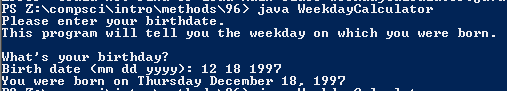# Assignment 96; WeekdayCalculator

## Code

```    // Donovan Rich
// 6
// Weekday Calculator
// WeekdayCalculator.java
// 3/24/2016

import java.util.Scanner;

public class WeekdayCalculator
{
public static void main( String[] args )
{
Scanner keyboard = new Scanner(System.in);

System.out.println("This program will tell you the weekday on which you were born.");
System.out.println();
System.out.print("Birth date (mm dd yyyy): ");
int month = keyboard.nextInt();
int day = keyboard.nextInt();
int year = keyboard.nextInt();

System.out.println("You were born on " + weekday(month,day,year));
}

public static String weekday( int month, int day, int year )
{
int total;
String date = "";
int yy = year - 1900;
total = (yy / 4) + yy + day;
total = monthOffset(month) + total;
boolean leapyear = isLeap(year);
if (leapyear == true )
{
if (month == 1 || month == 2 )
total = total - 1;

}

int weekday = total%7;
weekday_name(weekday);
date = weekday_name(weekday) + " " + monthName(month) + " " + day + ", " + year;

return date;
}

public static String monthName( int month)
{
String ans;
if ( month == 1 )
ans = "January";
else if ( month == 2 )
ans = "February";
else if ( month == 3 )
ans = "March";
else if ( month == 4 )
ans = "April";
else if ( month == 5 )
ans = "May";
else if ( month == 6 )
ans = "June";
else if ( month == 7 )
ans = "July";
else if ( month == 8 )
ans = "August";
else if ( month == 9 )
ans = "September";
else if ( month == 10 )
ans = "October";
else if ( month == 11 )
ans = "November";
else if ( month == 12 )
ans = "December";
else
ans = "Error";

return ans;
}
public static int monthOffset( int month )
{
int result;
if (month == 1)
result = 1;
else if (month == 2)
result = 4;
else if (month == 3)
result = 4;
else if (month == 4)
result = 0;
else if (month == 5)
result = 2;
else if (month == 6)
result = 5;
else if (month == 7)
result = 0;
else if (month == 8)
result = 3;
else if (month == 9)
result = 6;
else if (month == 10)
result = 1;
else if (month == 11)
result = 4;
else if (month == 12)
result = 6;
else
result = -1;
return result;
}
public static String weekday_name( int weekday )
{
String result = "";

if ( weekday == 1 )
{
result = "Sunday";
}
else if ( weekday == 2 )
{
result = "Monday";
}
else if(weekday == 3)
{
result = "Tuesday";
}
else if(weekday == 4)
{
result = "Wednseday";
}
else if(weekday == 5)
{
result = "Thursday";
}
else if(weekday == 6)
{
result = "Friday";
}
else if(weekday == 7)
{
result = "Saturday";
}
else if(weekday == 0)
{
result = "Saturday";
}
else
{
result = "error";
}
return result;

}

public static boolean isLeap( int year )
{
boolean result;

if ( year%400 == 0 )
{
result = true;
}
else if ( year%100 == 0 )
{
result = false;
}
else if ( year%4 == 0 )
{
result = true;
}
else
{
result = false;
}

return result;
}
}
```

### Picture of the output